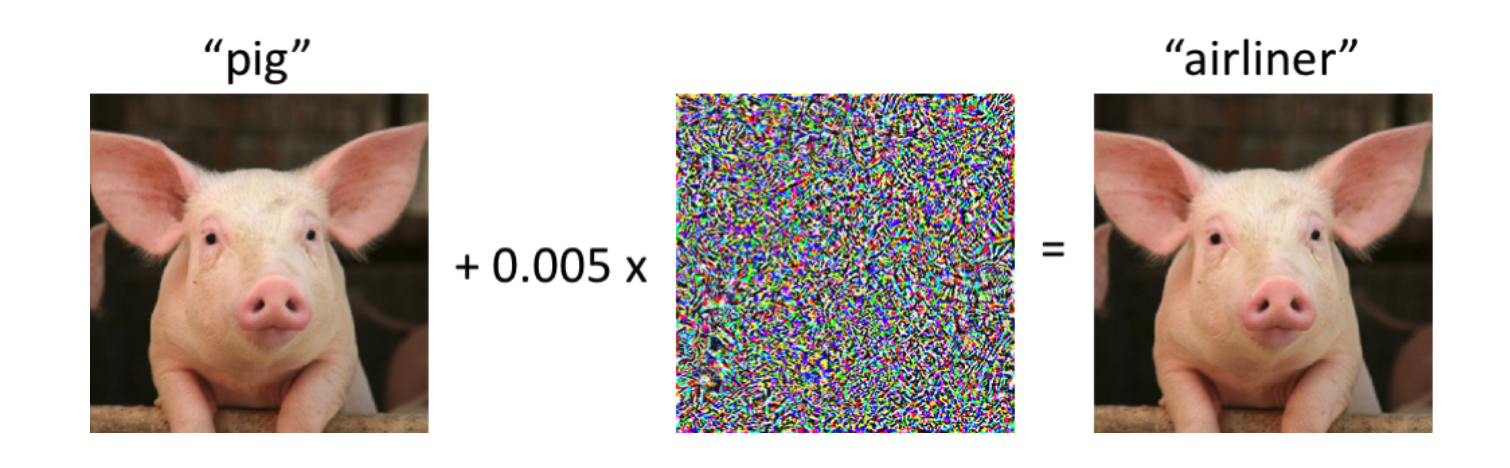# Investigations into the Complexity of Nonconvex Optimization

Machine learning based systems are set to play an increasing role in everyday life due to the increased abundance of large scale training data and the use of sophisticated statistical models such as neural networks. In light of these recent trends, much recent attention has been devoted towards understanding both how robust and reliable these methods are when deployed in the real world and the computational complexity of actually learning them from data. In our collaboration so far, we have adopted a theoretical perspective on each of these questions and have made partial progress towards understanding each of these phenomena in greater detail. We plan expand further on our theoretical explorations and empirically validate our findings in future work.

August 26, 2021

### Overview

We now overview each of the major themes of our collaboration. We start with our work on the susceptibility of neural networks to adversarial attacks before moving onto our work on understanding the complexity of training such networks.

#### Robustness

In the last few years, the robustness and reliability properties of machine learning based methods, particularly those based on neural networks, has been called into question due in part to the prevalence of adversarial examples. As these examples demonstrate, predictions made by these systems are often extremely brittle to the presence of even tiny, imperceptible perturbations to the input as illustrated in the following figure where a mild perturbation suffices to fool a state of the art machine learning based classifier.In our work so far, we undertook a theoretical exploration of when this phenomenon occurs in neural networks. This line of work was pioneered by Shamir et al  who showed that in most neural networks and most inputs, one can perturb a very small number of the entries of the input to cause the network to misclassify it. Noting that the sizes of these perturbations may be un-realistically large, Daniely and Schacham , showed that perturbations of much smaller magnitudes suffice to establish the existence of adversarial inputs. However, the main drawback of this approach is that it requires the network to be of rapidly decreasing width in each successive layer, an unnatural assumption in the context of neural network architectures used in practice. In two recent works, we remove this restrictive assumption on the widths of the networks. In our first result , we removed this assumption for a broad class of non-linearities in one-hidden-layer neural networks and substantially extended this result to the multi-layer setting in our second paper . Both of our results have since been accepted for publication at NeurIPS 2021.

A significant shortcoming of all prior work on this problem is that they only explain the existence of adversarial examples on random neural networks. In future work, we aim to explore the effects of training on the behavior of these networks. This is made challenging by the non-convexity of the optimization landscape encountered in the training of these networks. This problem remains open even in the simplest setting with just one hidden layer.

#### Complexity of Non-convex Optimization

A related question we pursued as part of our project was that of understanding the fundamental complexity of non-convex optimization. As previously noted, the problem of optimizing non-convex functions arises frequently in the training of deep learning systems. Despite its practical importance, optimal algorithms for even basic tasks in non-convex optimization remain poorly understood. This lies in stark contrast to the corresponding scenario for convex optimization where sharp rates are known in several regimes of interest.

In our project, we attempted to understand the complexity of the simple non-convex optimization problem of finding an approximate stationary point of a smooth non-convex function in terms of the number of queries to an oracle computing its value and gradient. Currently, the upper and lower bounds for this problem are only within polynomial factors of each other, leaving open the question of what the right complexity for this fundamental problem actually is. We studied the problem under the distributed setting where one is allowed to query the function several times during a single round with the parallelization would lead to faster optimization algorithms. Unfortunately, we showed that any distributed algorithm operating in fewer than polynomially many rounds must incur a complexity exponential in the dimension of the problem ruling out significant savings from parallelization. Given these results, the main question appears to be one of understanding the performance of a sequential algorithm, which is what we aim to characterize in future work.

### References

 Adi Shamir, Itay Safran, Eyal Ronen, and Orr Dunkelman. A simple explanation for the existence of adversarial examples with small hamming distance. CoRR, abs/1901.10861, 2019.

 Amit Daniely and Hadas Shacham. Most relu networks suffer from l2 adversarial perturbations. In Hugo Larochelle, Marc’Aurelio Ranzato, Raia Hadsell, Maria-Florina Balcan, and Hsuan-Tien Lin, editors, Advances in Neural Information Processing Systems 33: Annual Conference on Neural Information Processing Systems 2020, NeurIPS 2020, December 6-12, 2020, virtual, 2020

 Sébastien Bubeck, Yeshwanth Cherapanamjeri, Gauthier Gidel, and Rémi Tachet des Combes. A single gradient step finds adversarial examples on random two-layers neural networks.CoRR, abs/2104.03863,2021.

 Peter L. Bartlett, Sébastien Bubeck, and Yeshwanth Cherapanamjeri. Adversarial examples in multi-layer random relu networks. CoRR, abs/2106.12611, 2021